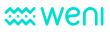# AlgorithmsUpdated by Evelyn Bernardo

There are three different algorithms on the Bothub: Statistical Model, Neural Network with Internal Vocabulary, Neural Network with External Vocabulary. You can choose which algorithm to use when you create a new bot or, if you want to change the algorithm after the creation, you can go to Settings and change it whenever you want. Each algorithm trains the resulting model in a different way and because of that, each one will provide different results.

##### Statistical Model

The Statistical Model uses pre-trained Word Embeddings and a simple statistical algorithm for classifying text in the selected language. The pre-trained Word Embeddings contain semantics and vocabulary information useful for the classification tasks. Each word in the vocabulary has a vector associated with them, and that helps the algorithm to calculate similarities between different words. The algorithm is able to recognize patterns in the vectors and even make operations such as sum words. Ex: Royals + Male = King or Royals + Female = Queen. So, when using the Statistical Model to analyze a sentence, Bothub will categorize it using similarity, which can result in false positives.

##### Neural Network with Internal Vocabulary

Neural Network with Internal Vocabulary uses only the vocabulary provided by the training set. Bothub attributes weights for each word, so every time you train your bot, this number is adjusted to fit de desired output. It is recommended to train at least 1000 sentences for each intent, and the more you train, more intelligent and precise your bot will be. In this example, we are using a binary bot that recognizes positive or negative, the analyzed sentence is “I don't think so”.

Bothub recognizes that it is more likely that this sentence has a negative intent because the higher confidence is negative:

{

"intent": {

"name": "negative",

"confidence": 0.5918083256887653

},

"intent_ranking": [{

"name": "negative",

"confidence": 0.5918083256887653

}, {

"name": "affirmative",

"confidence": 0.4081916743112346

}]

}

If the bot is not trained with enough sentences, Bothub might return no intent. It is relevant to say that the algorithm does not recognize the similarity between words, so for example, if you train Queen you will also need to train King, differently from the Statistical Model.

##### Neural Networking with External Vocabulary (BETA)

This algorithm makes use of the Statistical Model and Neural Network with Internal Vocabulary together. It uses Word Embeddings to attribute a pre-trained vector to each word, and a neural network to adjust weights in order to fit the desired output. As the Statistical Model, this algorithm does not return "no intent" so it will categorize the sentence within the most probable intent.

### How did we do?

#### Continuous improvements on your dataset